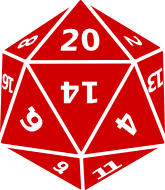The Five Platonic Solids

Math Lair Home > Topics > The Five Platonic Solids

Platonic solids, named after the Greek philosopher Plato, are three-dimensional solids whose polygonal surfaces are all the same (in math terms, congruent) and whose corners all meet at the same angles. Platonic solids are also referred to as regular polyhedra.

There are five and only five Platonic solids. They are: the cube, the tetrahedron, the octahedron, the dodecahedron, and the icosahedron. The properties of these solids are summarized in the following table:
NameFaceNumber of edges meeting at each vertexNumber of faces
TetrahedronTriangle34
OctahedronTriangle48
CubeSquare36
IcosahedronTriangle520
DodecahedronPentagon312

It can be shown using Euler's formula V + F = E + 2, which holds for any polyhedron, that there can only be five Platonic solids. In this formula, V is the number of vertices of the polyhedron, F the number of faces, and E the number of edges. In other words, the number of vertices of any polyhedron plus the number of faces is equal to the number of faces plus two.

Imposing the condition that all of the sides and angles be equal implies that there are only five Platonic solids. In order to have a polyhedron, at least three faces need to meet at each vertex and the sum of the angles of the faces meeting at each vertex must be less than 360° (if it were 360°, you would get a flat plane; a larger value is impossible). With this in mind, it isn't difficult to test out all possible solids to determine which meet the criteria. The angle of the face of a regular hexagon is 120°, so three would meet at a 360° angle, so no Platonic solid with hexagonal faces (or whose faces have more than six sides) is possible. For solids with a pentagonal face, the face angle is 108°, so three faces can meet at a vertex, giving a dodecahedron. Four would have a sum over 360°, which is impossible. For a square, the only possibility is for three faces to meet at a vertex, giving a cube. For a triangle, there are three possibilities (3, 4, or 5 faces meeting at each vertex, giving the tetrahedron, octahedron, and icosahedron).Here's an interesting practical application of Platonic solids. Since each of the sides are the same, they make good dice. If you happen to be a role-player (I'm not, though you'd never know it by just looking at these pages), have a look at the dice you use. You will probably have four-sided dice (tetrahedrons), six-sided dice (cubes), eight-sided dice (octahedrons), twelve-sided dice (dodecahedrons), and maybe even twenty-sided dice (icosahedrons), but most likely nothing else.